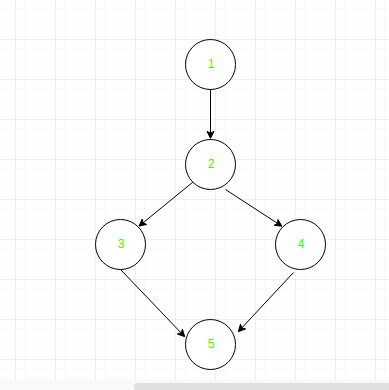# Data Flow Testing

• Difficulty Level : Easy
• Last Updated : 24 Oct, 2019

Data Flow Testing is a type of structural testing. It is a method that is used to find the test paths of a program according to the locations of definitions and uses of variables in the program. It has nothing to do with data flow diagrams.
It is concerned with:

• Statements where variables receive values,
• Statements where these values are used or referenced.

To illustrate the approach of data flow testing, assume that each statement in the program assigned a unique statement number. For a statement number S-

```DEF(S) = {X | statement S contains the definition of X}
USE(S) = {X | statement S contains the use of X} ```

If a statement is a loop or if condition then its DEF set is empty and USE set is based on the condition of statement s.

Data Flow Testing uses the control flow graph to find the situations that can interrupt the flow of the program.
Reference or define anomalies in the flow of the data are detected at the time of associations between values and variables. These anomalies are:

• A variable is defined but not used or referenced,
• A variable is used but never defined,
• A variable is defined twice before it is used

Data Flow Testing is used to find the following issues-

• To find a variable that is used but never defined,
• To find a variable that is defined but never used,
• To find a variable that is defined multiple times before it is use,
• Deallocating a variable before it is used.

• Time consuming and costly process
• Requires knowledge of programming languages

Example:

```1. read x, y;
2. if(x>y)
3. a = x+1
else
4. a = y-1
5. print a; ```

Control flow graph of above example:Define/use of variables of above example:

VariableDefined at nodeUsed at node
x12, 3
y12, 4
a3, 45

My Personal Notes arrow_drop_up